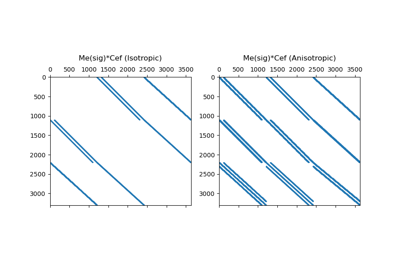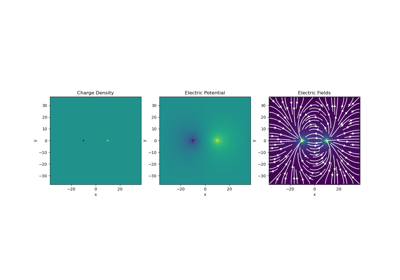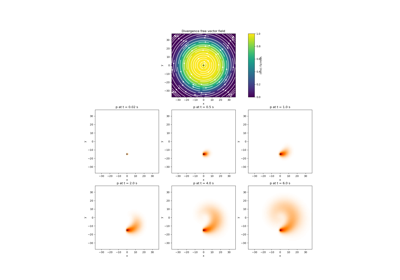# discretize.TensorMesh#class discretize.TensorMesh(h, origin=None, **kwargs)[source]#

Tensor mesh class.

Tensor meshes are numerical grids whose cell centers, nodes, faces, edges, widths, volumes, etc… can be directly expressed as tensor products. The axes defining coordinates of the mesh are orthogonal. And cell properties along one axis do not vary with respect to the position along any other axis.

Parameters
h(dim) iterable of int, numpy.ndarray, or tuple

Defines the cell widths along each axis. The length of the iterable object is equal to the dimension of the mesh (1, 2 or 3). For a 3D mesh, the list would have the form [hx, hy, hz] .

Along each axis, the user has 3 choices for defining the cells widths:

• int -> A unit interval is equally discretized into N cells.

• numpy.ndarray -> The widths are explicity given for each cell

• the widths are defined as a list of tuple of the form (dh, nc, [npad]) where dh is the cell width, nc is the number of cells, and npad (optional) is a padding factor denoting exponential increase/decrease in the cell width for each cell; e.g. [(2., 10, -1.3), (2., 50), (2., 10, 1.3)]

origin(dim) iterable, default: 0

Define the origin or ‘anchor point’ of the mesh; i.e. the bottom-left-frontmost corner. By default, the mesh is anchored such that its origin is at [0, 0, 0] .

For each dimension (x, y or z), The user may set the origin 2 ways:

• a scalar which explicitly defines origin along that dimension.

• {‘0’, ‘C’, ‘N’} a str specifying whether the zero coordinate along each axis is the first node location (‘0’), in the center (‘C’) or the last node location (‘N’) (see Examples).

utils.unpack_widths

The function used to expand a tuple to generate widths.

Examples

An example of a 2D tensor mesh is shown below. Here we use a list of tuple to define the discretization along the x-axis and a numpy array to define the discretization along the y-axis. We also use a string argument to center the x-axis about x = 0 and set the top of the mesh to y = 0.

>>> from discretize import TensorMesh
>>> import matplotlib.pyplot as plt

>>> ncx = 10      # number of core mesh cells in x
>>> dx = 5        # base cell width x
>>> exp_x = 1.25  # expansion rate of padding cells in x
>>> ncy = 24      # total number of mesh cells in y
>>> dy = 5        # base cell width y

>>> hx = [(dx, npad_x, -exp_x), (dx, ncx), (dx, npad_x, exp_x)]
>>> hy = dy * np.ones(ncy)
>>> mesh = TensorMesh([hx, hy], origin='CN')

>>> fig = plt.figure(figsize=(5,5))
>>> mesh.plot_grid(ax=ax)
>>> plt.show()


Attributes

 area area has been deprecated. areaFx areaFx has been deprecated. areaFy areaFy has been deprecated. areaFz areaFz has been deprecated. average_cell_to_edge Averaging operator from cell centers to edges (scalar quantities). average_cell_to_face Averaging operator from cell centers to faces (scalar quantities). average_cell_vector_to_face Averaging operator from cell centers to faces (vector quantities). average_edge_to_cell Averaging operator from edges to cell centers (scalar quantities). average_edge_to_cell_vector Averaging operator from edges to cell centers (vector quantities). average_edge_to_face_vector Averaging operator from edges to faces (vector quantities). average_edge_x_to_cell Averaging operator from x-edges to cell centers (scalar quantities). average_edge_y_to_cell Averaging operator from y-edges to cell centers (scalar quantities). average_edge_z_to_cell Averaging operator from z-edges to cell centers (scalar quantities). average_face_to_cell Averaging operator from faces to cell centers (scalar quantities). average_face_to_cell_vector Averaging operator from faces to cell centers (vector quantities). average_face_x_to_cell Averaging operator from x-faces to cell centers (scalar quantities). average_face_y_to_cell Averaging operator from y-faces to cell centers (scalar quantities). average_face_z_to_cell Averaging operator from z-faces to cell centers (scalar quantities). average_node_to_cell Averaging operator from nodes to cell centers (scalar quantities). average_node_to_edge Averaging operator from nodes to edges (scalar quantities). average_node_to_face Averaging operator from nodes to faces (scalar quantities). axis_u Orientation of the first axis. axis_v Orientation of the second axis. axis_w Orientation of the third axis. boundary_edge_vector_integral Integrate a vector function on the boundary. boundary_edges Boundary edge locations. boundary_face_outward_normals Outward normal vectors of boundary faces. boundary_face_scalar_integral Represent the operation of integrating a scalar function on the boundary. boundary_faces Boundary face locations. boundary_node_vector_integral Integrate a vector function dotted with the boundary normal. boundary_nodes Boundary node locations. cellBoundaryInd cellBoundaryInd has been deprecated. cellGrad cellGrad has been deprecated. cellGradBC cellGradBC has been deprecated. cellGradx cellGradx has been deprecated. cellGrady cellGrady has been deprecated. cellGradz cellGradz has been deprecated. cell_boundary_indices Return the indices of the x, (y and z) boundary cells. cell_centers Return gridded cell center locations. cell_centers_x Return x-coordinates of the cell centers along the x-direction. cell_centers_y Return y-coordinates of the cell centers along the y-direction. cell_centers_z Return z-coordinates of the cell centers along the z-direction. cell_gradient Cell gradient operator (cell centers to faces). cell_gradient_BC Boundary conditions matrix for the cell gradient operator (Deprecated). cell_gradient_x X-derivative operator (cell centers to x-faces). cell_gradient_y Y-derivative operator (cell centers to y-faces). cell_gradient_z Z-derivative operator (cell centers to z-faces). cell_volumes Return cell volumes. dim The dimension of the mesh (1, 2, or 3). edge edge has been deprecated. edgeCurl edgeCurl has been deprecated. edgeEx edgeEx has been deprecated. edgeEy edgeEy has been deprecated. edgeEz edgeEz has been deprecated. edge_curl Edge curl operator (edges to faces). edge_lengths Return lengths of all edges in the mesh. edge_tangents Unit tangent vectors for all mesh edges. edge_x_lengths Return the x-edge lengths. edge_y_lengths Return the y-edge lengths. edge_z_lengths Return the z-edge lengths. edges Gridded edge locations. edges_x Gridded x-edge locations. edges_y Gridded y-edge locations. edges_z Gridded z-edge locations. faceBoundaryInd faceBoundaryInd has been deprecated. faceDiv faceDiv has been deprecated. faceDivx faceDivx has been deprecated. faceDivy faceDivy has been deprecated. faceDivz faceDivz has been deprecated. face_areas Return areas of all faces in the mesh. face_boundary_indices Return the indices of the x, (y and z) boundary faces. face_divergence Face divergence operator (faces to cell-centres). face_normals Unit normal vectors for all mesh faces. face_x_areas Return the areas of the x-faces. face_x_divergence X-derivative operator (x-faces to cell-centres). face_y_areas Return the areas of the y-faces. face_y_divergence Y-derivative operator (y-faces to cell-centres). face_z_areas Return the areas of the z-faces. face_z_divergence Z-derivative operator (z-faces to cell-centres). faces Gridded face locations. faces_x Gridded x-face locations. faces_y Gridded y-face locations. faces_z Gridded z-face locations. h Cell widths along each axis direction. h_gridded Return dimensions of all mesh cells as staggered grid. hx Width of cells in the x direction. hy Width of cells in the y direction. hz Width of cells in the z direction. nCx Number of cells in the x direction. nCy Number of cells in the y direction. nCz Number of cells in the z direction. nNx Number of nodes in the x-direction. nNy Number of nodes in the y-direction. nNz Number of nodes in the z-direction. n_cells Total number of cells in the mesh. n_edges Total number of edges in the mesh. n_edges_per_direction The number of edges in each direction. n_edges_x Number of x-edges in the mesh. n_edges_y Number of y-edges in the mesh. n_edges_z Number of z-edges in the mesh. n_faces Total number of faces in the mesh. n_faces_per_direction The number of faces in each axis direction. n_faces_x Number of x-faces in the mesh. n_faces_y Number of y-faces in the mesh. n_faces_z Number of z-faces in the mesh. n_nodes Total number of nodes in the mesh. nodalGrad nodalGrad has been deprecated. nodalLaplacian nodalLaplacian has been deprecated. nodal_gradient Nodal gradient operator (nodes to edges). nodal_laplacian Nodal scalar Laplacian operator (nodes to nodes). nodes Return gridded node locations. nodes_x Return x-coordinates of the nodes along the x-direction. nodes_y Return y-coordinates of the nodes along the y-direction. nodes_z Return z-coordinates of the nodes along the z-direction. normals normals has been deprecated. orientation Rotation matrix defining mesh axes relative to Cartesian. origin Origin or 'anchor point' of the mesh. project_edge_to_boundary_edge Projection matrix from all edges to boundary edges. project_face_to_boundary_face Projection matrix from all faces to boundary faces. project_node_to_boundary_node Projection matrix from all nodes to boundary nodes. reference_is_rotated Indicate whether mesh uses standard coordinate axes. reference_system Coordinate reference system. rotation_matrix Alias for orientation. shape_cells Number of cells in each coordinate direction. shape_edges_x Number of x-edges along each axis direction. shape_edges_y Number of y-edges along each axis direction. shape_edges_z Number of z-edges along each axis direction. shape_faces_x Number of x-faces along each axis direction. shape_faces_y Number of y-faces along each axis direction. shape_faces_z Number of z-faces along each axis direction. shape_nodes The number of nodes along each axis direction. stencil_cell_gradient Stencil for cell gradient operator (cell centers to faces). stencil_cell_gradient_x Differencing operator along x-direction (cell centers to x-faces). stencil_cell_gradient_y Differencing operator along y-direction (cell centers to y-faces). stencil_cell_gradient_z Differencing operator along z-direction (cell centers to z-faces). tangents tangents has been deprecated. vectorCCx vectorCCx has been deprecated. vectorCCy vectorCCy has been deprecated. vectorCCz vectorCCz has been deprecated. vectorNx vectorNx has been deprecated. vectorNy vectorNy has been deprecated. vectorNz vectorNz has been deprecated. vol vol has been deprecated. x0 Alias for the origin.

Methods

 cell_gradient_weak_form_robin([alpha, beta, ...]) Create Robin conditions pieces for weak form of the cell gradient operator (cell centers to faces). closest_points_index(locations[, grid_loc, ...]) Find the indicies for the nearest grid location for a set of points. Make a copy of the current mesh. deserialize(items, **kwargs) Create this mesh from a dictionary of attributes. edge_divergence_weak_form_robin([alpha, ...]) Create Robin conditions pieces for weak form of the edge divergence operator (edges to nodes). equals(other_mesh) Compare the current mesh with another mesh to determine if they are identical. from_omf(element) Convert an omf object to a discretize mesh. getBCProjWF(*args, **kwargs) getBCProjWF has been deprecated. getBCProjWF_simple(*args, **kwargs) getBCProjWF_simple has been deprecated. getEdgeInnerProduct(*args, **kwargs) getEdgeInnerProduct has been deprecated. getEdgeInnerProductDeriv(*args, **kwargs) getEdgeInnerProductDeriv has been deprecated. getFaceInnerProduct(*args, **kwargs) getFaceInnerProduct has been deprecated. getFaceInnerProductDeriv(*args, **kwargs) getFaceInnerProductDeriv has been deprecated. getInterpolationMat(*args, **kwargs) getInterpolationMat has been deprecated. getTensor(*args, **kwargs) getTensor has been deprecated. get_BC_projections(BC[, discretization]) Create the weak form boundary condition projection matrices. get_BC_projections_simple([discretization]) Create weak form boundary condition projection matrices for mixed boundary condition. get_edge_inner_product([model, ...]) Generate the edge inner product matrix or its inverse. get_edge_inner_product_deriv(model[, ...]) Get a function handle to multiply vector with derivative of edge inner product matrix (or its inverse). get_face_inner_product([model, ...]) Generate the face inner product matrix or its inverse. get_face_inner_product_deriv(model[, ...]) Get a function handle to multiply a vector with derivative of face inner product matrix (or its inverse). get_interpolation_matrix(loc[, ...]) Construct a linear interpolation matrix from mesh. get_tensor(key) Return the base 1D arrays for a specified mesh tensor. isInside(*args, **kwargs) isInside has been deprecated. is_inside(pts[, location_type]) Determine which points lie within the mesh. plotGrid(*args, **kwargs) plotGrid has been deprecated. plotImage(*args, **kwargs) plotImage has been deprecated. plotSlice(*args, **kwargs) plotSlice has been deprecated. plot_3d_slicer(v[, xslice, yslice, zslice, ...]) Plot slices of a 3D volume, interactively (scroll wheel). plot_grid([ax, nodes, faces, centers, ...]) Plot the grid for nodal, cell-centered and staggered grids. plot_image(v[, v_type, grid, view, ax, ...]) Plot quantities defined on a given mesh. plot_slice(v[, v_type, normal, ind, ...]) Plot a slice of fields on the given 3D mesh. point2index(locs) Find cells that contain the given points. projectEdgeVector(*args, **kwargs) projectEdgeVector has been deprecated. projectFaceVector(*args, **kwargs) projectFaceVector has been deprecated. project_edge_vector(edge_vectors) Project vectors to the edges of the mesh. project_face_vector(face_vectors) Project vectors onto the faces of the mesh. r(*args, **kwargs) r has been deprecated. readModelUBC(*args, **kwargs) readModelUBC has been deprecated. readUBC(file_name[, directory]) Read 2D or 3D tensor mesh from UBC-GIF formatted file. readVTK(file_name[, directory]) Read VTK rectilinear file (vtr or xml) and return a discretize tensor mesh (and models). read_UBC(file_name[, directory]) Read 2D or 3D tensor mesh from UBC-GIF formatted file. read_model_UBC(file_name[, directory]) Read UBC-GIF formatted model file for 2D or 3D tensor mesh. read_vtk(file_name[, directory]) Read VTK rectilinear file (vtr or xml) and return a discretize tensor mesh (and models). reshape(x[, x_type, out_type, return_format]) Reshape tensor quantities. save([file_name, verbose]) Save the mesh to json. Represent the mesh's attributes as a dictionary. setCellGradBC(*args, **kwargs) setCellGradBC has been deprecated. Set boundary conditions for derivative operators acting on cell-centered quantities. toVTK([models]) Convert mesh (and models) to corresponding VTK or PyVista data object. Represent the mesh's attributes as a dictionary. to_omf([models]) Convert to an omf data object. to_vtk([models]) Convert mesh (and models) to corresponding VTK or PyVista data object. Return the validation state of the mesh. vtk_to_tensor_mesh(vtrGrid) Convert vtk object to a TensorMesh. writeModelUBC(*args, **kwargs) writeModelUBC has been deprecated. writeUBC(*args, **kwargs) writeUBC has been deprecated. writeVTK(file_name[, models, directory]) Convert mesh (and models) to corresponding VTK or PyVista data object then writes to file. write_UBC(file_name[, models, directory, ...]) Write 2D or 3D tensor mesh (and models) to UBC-GIF formatted file(s). write_model_UBC(file_name, model[, directory]) Write 2D or 3D tensor model to UBC-GIF formatted file. write_vtk(file_name[, models, directory]) Convert mesh (and models) to corresponding VTK or PyVista data object then writes to file.

## Galleries and Tutorials using discretize.TensorMesh#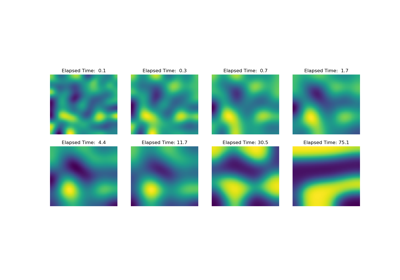Operators: Cahn Hilliard

Operators: Cahn Hilliard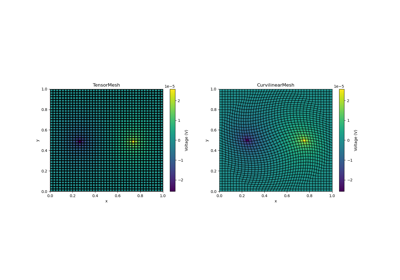Basic Forward 2D DC Resistivity

Basic Forward 2D DC ResistivityBasic: PlotImage

Basic: PlotImage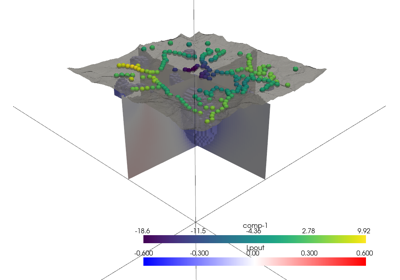3D Visualization with PyVista

3D Visualization with PyVista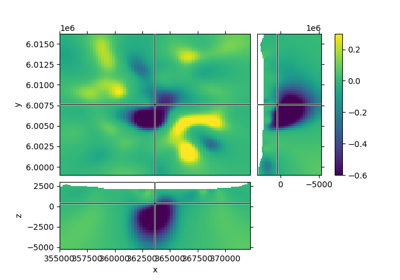Slicer demo

Slicer demo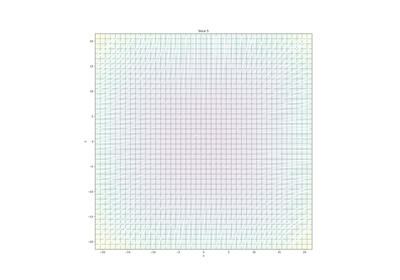Plotting: Streamline thickness

Plotting: Streamline thickness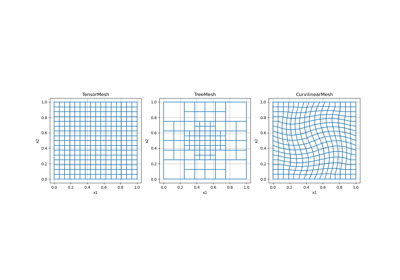Overview of Mesh Types

Overview of Mesh Types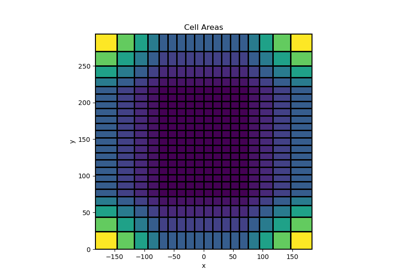Tensor meshes

Tensor meshes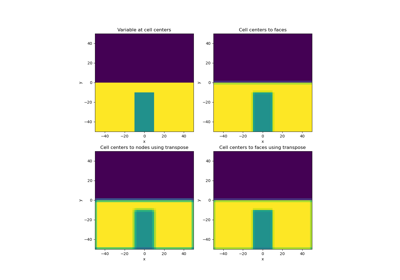Averaging Matricies

Averaging Matricies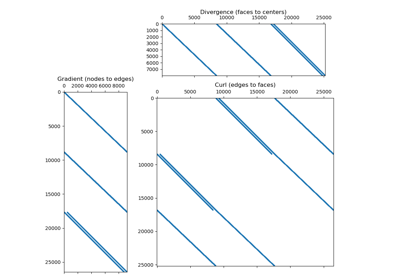Differential Operators

Differential Operators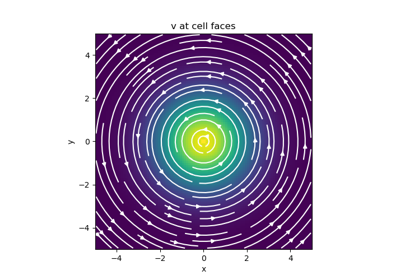Basic Inner Products

Basic Inner Products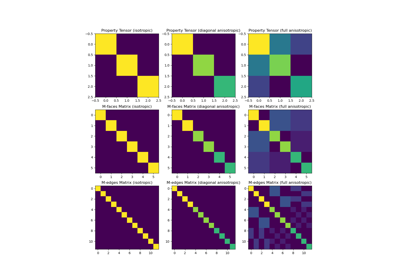Constitutive Relations

Constitutive Relations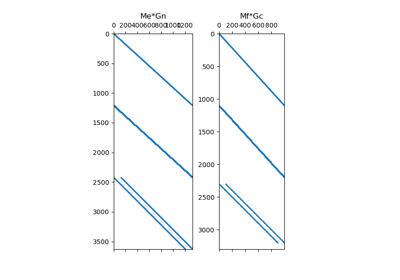Differential Operators

Differential Operators